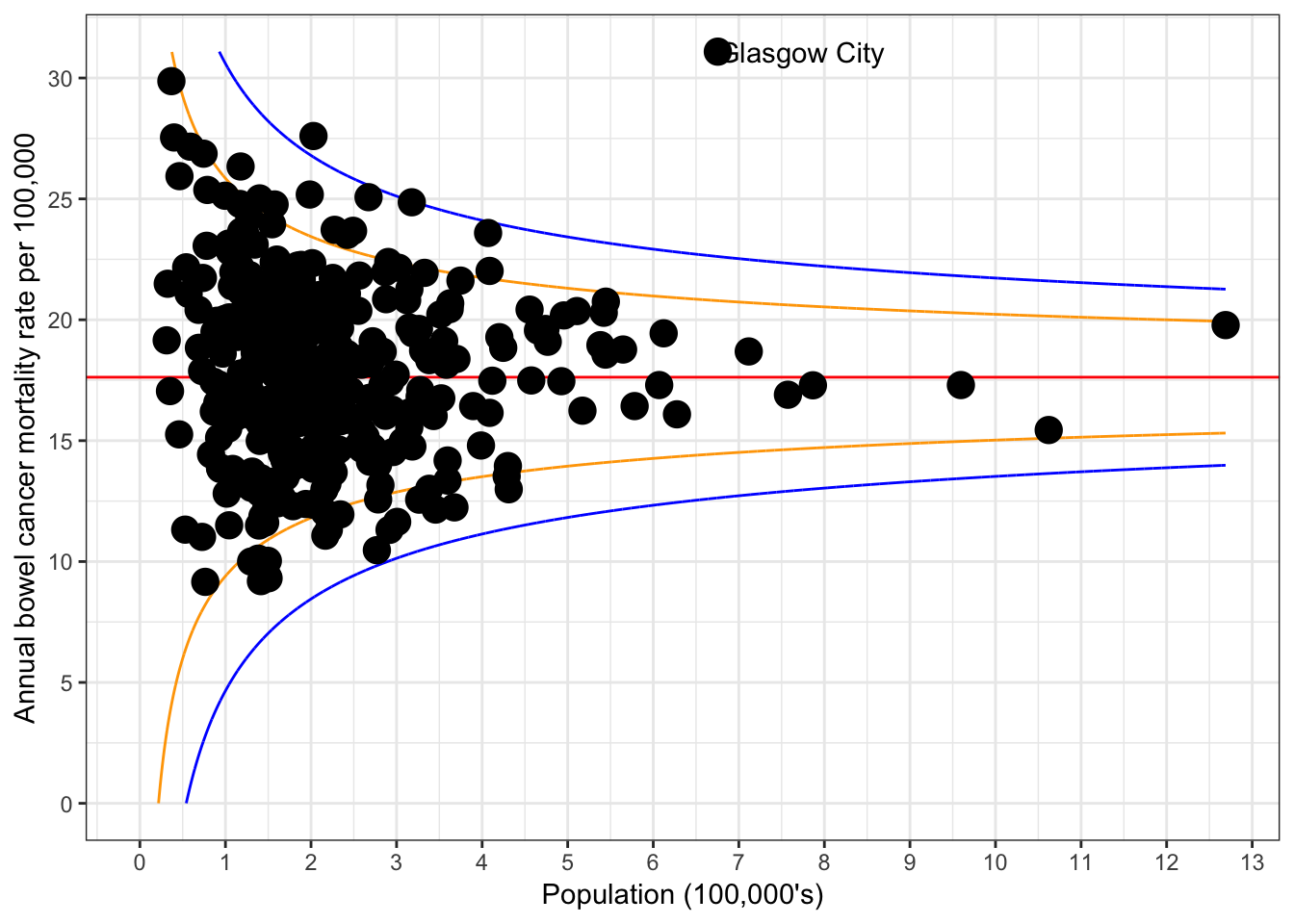The date are discussed in a blog by Paul Barden on the Understanding Uncertainty site

and on his own blog. Note that data for Wales is not included, as it was reported for the whole of Wales rather than by region.

library(ggplot2)

summary(bowel.data)
##               Country                District         n
##  England          :324   Aberdeen City   :  1   Min.   :  6.0
##  Northern Ireland : 24   Aberdeenshire   :  1   1st Qu.: 24.0
##  Scotland         : 31   Angus           :  1   Median : 32.0
##                          Antrim          :  1   Mean   : 39.6
##                          Ards            :  1   3rd Qu.: 45.0
##                          Argyll and Bute :  1   Max.   :251.0
##                          (Other)         :373
##        d
##  Min.   :  31332
##  1st Qu.: 140110
##  Median : 189202
##  Mean   : 224700
##  3rd Qu.: 267794
##  Max.   :1268959
##
attach(bowel.data)
mean.prop=sum(n)/sum(d)
props=n/d
max.props=max(props)

# try funnelR package
library(funnelR)

# Numerator must be called n, denomnator d
funnel_limits   <- fundata(input=bowel.data,benchmark=mean.prop, alpha=0.95, alpha2=0.998, method='approximate', step=100)

funnel_plot     <- funplot(input=bowel.data, fundata=funnel_limits)

funnel_plot = funnel_plot + coord_cartesian(ylim = c(0,max.props) )
## Coordinate system already present. Adding new coordinate system, which will replace the existing one.
#funnel_plot = funnel_plot + geom_hline(yintercept=mean.prop, colour="darkred", linetype=6, size=1)
funnel_plot = funnel_plot + scale_x_continuous(name="Population (100,000's)", breaks=100000*(0:14), labels=0:14, limits=c(0,max(d)))
funnel_plot = funnel_plot + scale_y_continuous(name="Annual bowel cancer mortality rate per 100,000", breaks=5*(0:8)/100000, labels=5*(0:8), limits=c(0,max.props))
## Scale for 'y' is already present. Adding another scale for 'y', which
## will replace the existing scale.
glasgow <- subset(bowel.data, District == "Glasgow City")  # identify Glasgow City in data frame

#funnel_plot = funnel_plot + geom_text(data=glasgow, label="Glasgow City", vjust=1)

funnel_plot = funnel_plot + annotate("text", x=glasgow$d,y=glasgow$n/glasgow\$d,label="Glasgow City",hjust=0, vjust=0.5)

funnel_plot
## Warning: Removed 374 rows containing missing values (geom_path).
## Warning: Removed 218 rows containing missing values (geom_path).
## Warning: Removed 928 rows containing missing values (geom_path).
## Warning: Removed 542 rows containing missing values (geom_path).